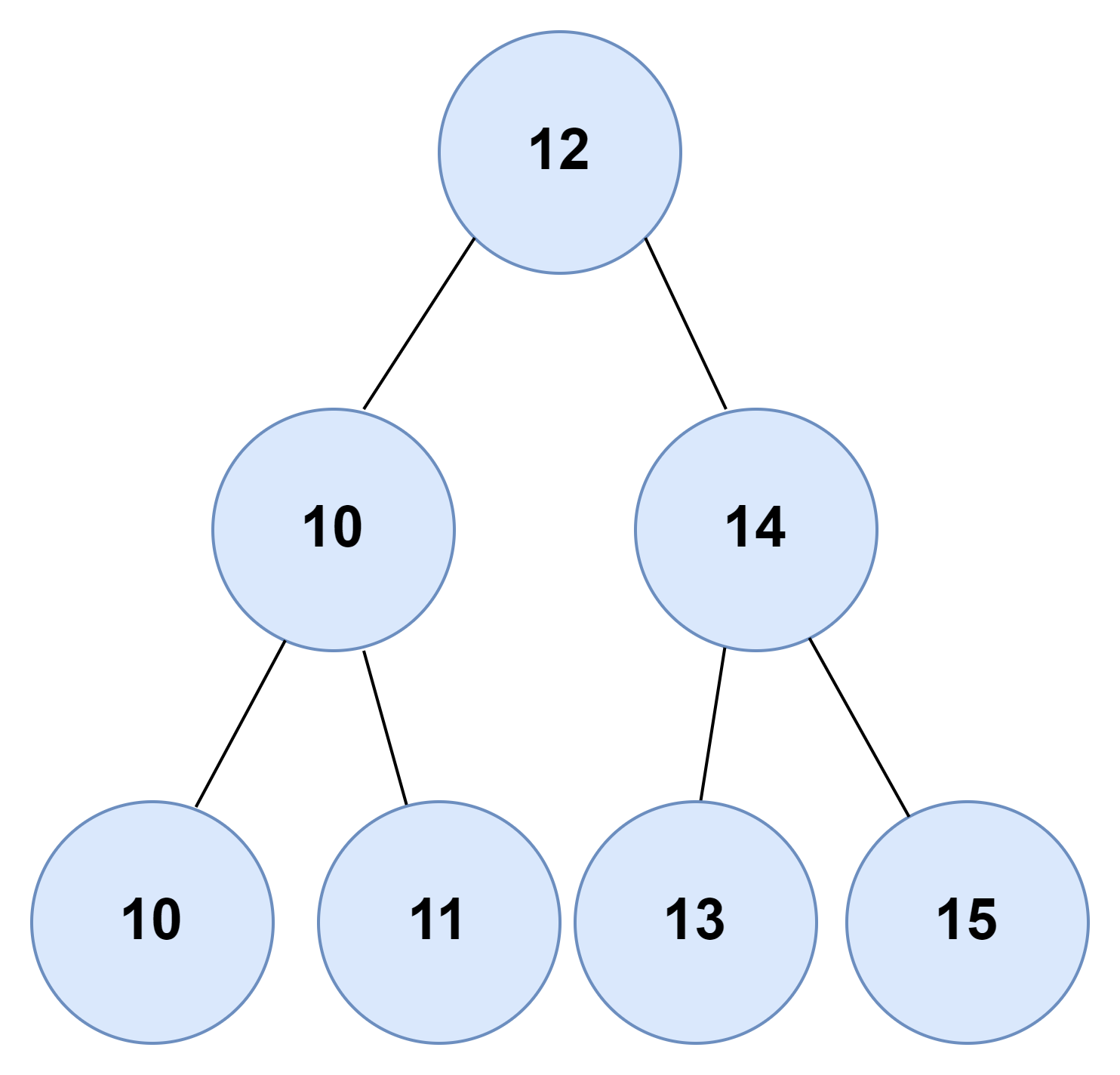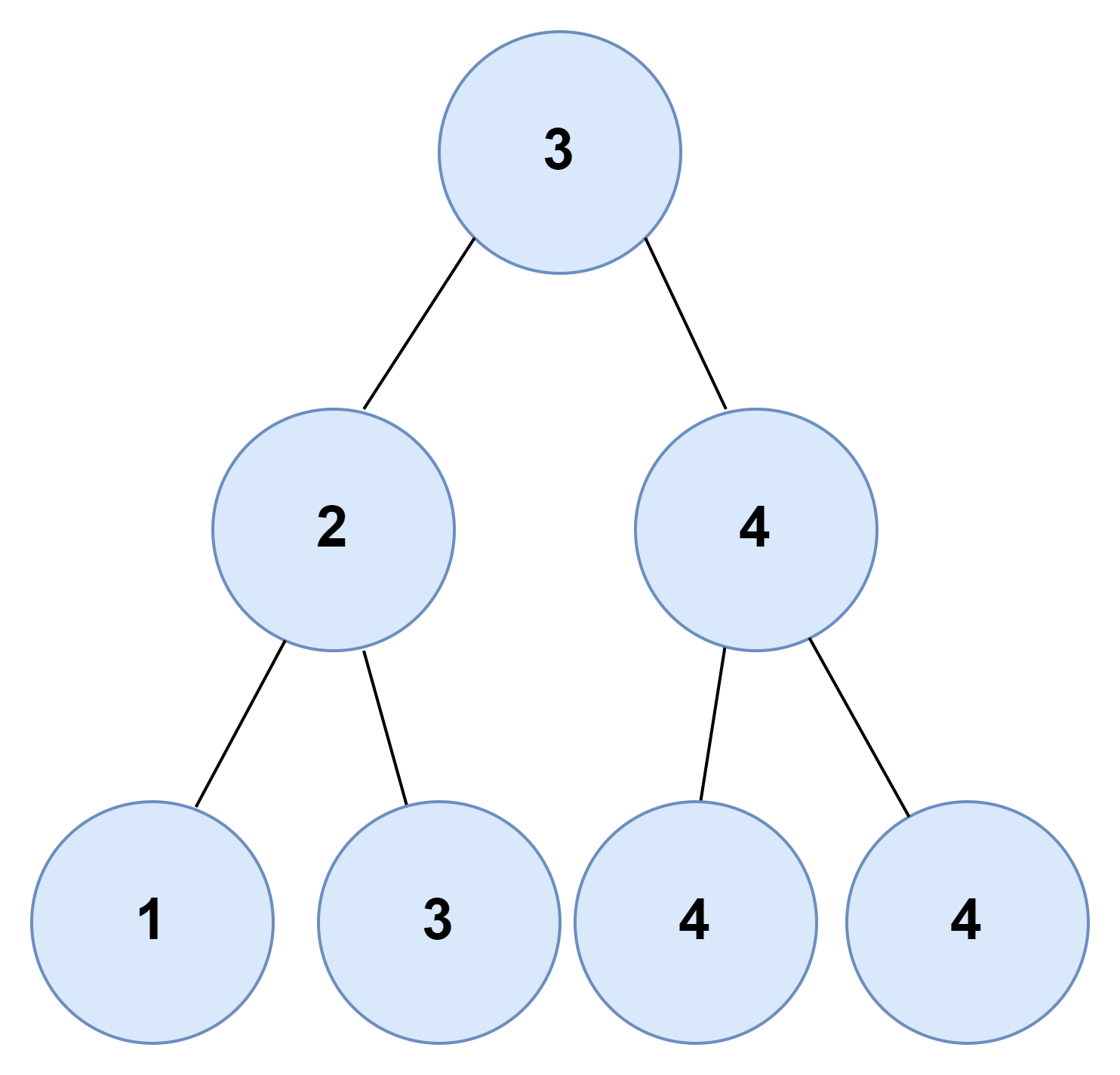# Find the maximum count of duplicate nodes in a Binary Search Tree

## Introduction

This blog will discuss the solution to the problem to find the maximum count of duplicate nodes in a binary search tree, here we are given a binary search tree. It has duplicate nodes, and we need to find the maximum count of duplicate nodes in this binary search tree. If there is more than one node with a maximum count, we can print any one of them.

### Sample Examples

Input: The given bst:Output: The node with a maximum count of duplicates is: 10

Input: The given bst:Output: The node with a maximum count of duplicates is: 4

## Brute Force Approach

The brute force approach of this problem to find the maximum count of duplicate nodes in a Binary Search Tree is to hash all the node values of the bst in the map.

After that, we will traverse the map and store the node with the maximum hash value in a variable because the hash value equals the count of nodes in the bst.

### Algorithm

1. We will make a function inorder() which will take one argument, i.e., the tree's root. We will maintain a map and hash all the elements of the bst into the map while traversing the bst.
2. Now in the main code, we will traverse the map and maintain a variable to store the Node with the maximum count of duplicates.
3. After the map iteration is over, we will print our answer.

### Code in C++

``````// C++ implementation to find the maximum count of duplicate nodes in a binary search tree
#include <bits/stdc++.h>
using namespace std;

// Structure of each node of BST
struct Node
{
int key;
struct Node *left, *right;
};

// Function to create a new BST node
Node *TreeNode(int data)
{
Node *temp = new Node();
temp->key = data;
temp->left = temp->right = NULL;
return temp;
}

// Function to insert node in bst
struct Node *insert(struct Node *node, int key)
{
// If the tree is empty, then return a new node
if (node == NULL)
return TreeNode(key);

// Otherwise, recur down the tree
if (key <= node->key)
node->left = insert(node->left, key);
else if (key > node->key)
node->right = insert(node->right, key);

// Return the (unchanged) node pointer
return node;
}

// to hash the elements of the bst
map<int, int> m;

// Function to count of duplicate nodes in a bst
void inorder(struct Node *root)
{
// base case
if (!root)
return;

// hashing the current node in the map
m[root->key]++;

inorder(root->left);

inorder(root->right);
}
int main()
{

/* Formation of BST 1
5
/ \
2   7
/\   /\
1  4 6  10
*/

struct Node *root1 = NULL;
root1 = insert(root1, 5);
insert(root1, 2);
insert(root1, 2);
insert(root1, 4);
insert(root1, 7);
insert(root1, 6);
insert(root1, 10);

int x = 7, ans = 0, node = 0;
inorder(root1);
for (auto y : m)
{
// to check for node with maximum count of duplicates
if (ans < y.second)
{
ans = max(ans, y.second);
node = y.first;
}
}
cout << "The node with a maximum count of duplicates is:" << node << endl;

return 0;
}``````

Output:

``The node with a maximum count of duplicates is: 2``

#### Complexity Analysis

Time Complexity: O(N)

Since we are doing inorder traversal of the bst and inorder traversal can be done in O(N), the time complexity is O(N).

Space Complexity: O(N)

Since we are hashing all the nodes in the map, therefore, the space complexity is O(N).

## Optimized Approach

Find the maximum count of duplicate nodes in a binary search tree to solve this problem. We need to find the inorder traversal of the bst because it will give us the nodes of the tree in sorted order, so the idea to solve this problem is simple we will do recursive inorder traversal, and we will keep track of the previous node value, and if the previous node value is equal to the current node value then we will increase the count, and if the count is greater than maximum count then we will replace maximum count with the current count.

### Algorithm

1. We will make a function inorder() which will take one argument, i.e., the tree's root. We will also maintain four variables, two for counting duplicates, one for storing the answer value, and the last one for storing the previous node.
2. We will do recursive inorder traversal, keeping track of the previous node. If the value of the previous node is equal to the current node's value, then we will increase the count. If the count value becomes greater than the maximum count value, we will replace the maximum value and simultaneously assign the answer with the root node's value.
3. We will now call this function in main, and after that, we will print the answer variable.

### Code in C++

``````// C++ implementation to find the maximum count of duplicate nodes in a binary search tree
#include <bits/stdc++.h>
using namespace std;

// Structure of each node of BST
struct Node
{
int key;
struct Node *left, *right;
};

// Function to create a new BST node
Node *TreeNode(int data)
{
Node *temp = new Node();
temp->key = data;
temp->left = temp->right = NULL;
return temp;
}

// Function to insert node in bst
struct Node *insert(struct Node *node, int key)
{
// If the tree is empty, then return a new node
if (node == NULL)
return TreeNode(key);

// Otherwise, recur down the tree
if (key < node->key)
node->left = insert(node->left, key);
else if (key > node->key)
node->right = insert(node->right, key);

// Return the (unchanged) node pointer
return node;
}

// max_count to store the value of maximum count till now
// count_for_duplicates to store current count value
// and answer to store the node having maximum duplicates
int max_count = 0, count_for_duplicates = 1, answer;

struct Node *previous = NULL;

// Function to find the maximum count of duplicate nodes in a binary search tree
void inorder(struct Node *root)
{
// base case
if (!root)
{
return;
}
inorder(root->left);
if (previous != NULL)
{
// if previous value is equal to root value
// then increase variable count_for_duplicates
if (previous->key == root->key)
{
count_for_duplicates++;
}
// else reset its value to one
else
{
count_for_duplicates = 1;
}
}
// if the variable count_for_duplicates has more value than
// the maximum count value then replace the maximum count value
// and assign answer the current node's value
if (count_for_duplicates > max_count)
{
max_count = count_for_duplicates;
}
// assign previous the value of current node
previous = root;
inorder(root->right);
}
int main()
{

/* Formation of BST
5
/ \
3   7
/\   /\
3  3 6  8
*/

struct Node *root = NULL;
root1 = insert(root, 5);
insert(root, 3);
insert(root, 3);
insert(root, 3);
insert(root, 7);
insert(root, 6);
insert(root, 8);

inorder(root);

cout << "The node with a maximum count of duplicates is: " << answer << endl;

return 0;
}``````

Output:

``The node with a maximum count of duplicates is: 3 ``

#### Complexity Analysis

Time Complexity: O(N)

Since we are doing inorder traversal of the bst and inorder traversal can be done in O(N), the time complexity is O(N).

Space Complexity: O(1)

If we ignore the stack space occupied by the recursive calls, the space complexity will be O(1).

Q1. What is a binary tree?

Ans. A binary tree is a data structure in programming languages to represent the hierarchy.  Each node of the binary tree can have either 0,1, or 2 children.

Q2. What are leave nodes in a tree?

Ans. Leave nodes of a tree are those nodes that don’t have any children, and both the pointers of the nodes point to null.

Q3. What is a binary search tree?

Ans. A binary search tree is a sorted binary tree in which all the nodes of the binary tree have a value greater than their left child node and a value smaller than their right child node.

## Key takeaways

In this article, we discussed the problem find the maximum count of duplicate nodes in a binary search tree, and we have discussed its most efficient approach. We hope you have gained a better understanding of the solution to this problem and, now it is your responsibility to solve the problem and try some new and different approaches to this problem.

You can learn more about binary search trees hereUntil then, Keep Learning and Keep Coding and practicing on Code studio.